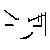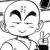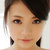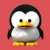# PHP 使用协同程序实现合作多任务已翻译 100%

oschina 投递于 2013/06/20 22:30 (共 20 段, 翻译完成于 06-29)

#### 参与翻译 (5人) : 几点人, K6F, TigaPile, 杨少瑜, 蒋锴

PHP5.5一个比较好的新功能是实现对生成器和协同程序的支持。对于生成器，PHP的文档和各种其他的博客文章（就像这一个这一个）已经有了非常详细的讲解。协同程序相对受到的关注就少了，所以协同程序虽然有很强大的功能但也很难被知晓，解释起来也比较困难。

### 生成器

<?php

```<?php

function xrange(\$start, \$end, \$step = 1) {
for (\$i = \$start; \$i <= \$end; \$i += \$step) {
yield \$i;
}
}

foreach (xrange(1, 1000000) as \$num) {
echo \$num, "\n";
}
```

### 生成器为可中断的函数

```<?php

\$range = xrange(1, 1000000);
var_dump(\$range); // object(Generator)#1
var_dump(\$range instanceof Iterator); // bool(true)
```

你对某个对象调用迭代器方法一次，其中的代码运行一次。例如，如果你调用\$range->rewind(),那么xrange()里的代码运行到控制流 第一次出现yield的地方。在这种情况下，这就意味着当\$i=\$start时yield \$i才运行。传递给yield语句的值是使用\$range->current()获取的。

为了继续执行生成器中的代码，你必须调用\$range->next()方法。这将再次启动生成器，直到yield语句出现。因此，连续调用next()和current()方法 你将能从生成器里获得所有的值，直到某个点没有再出现yield语句。对xrange()来说，这种情形出现在\$i超过\$end时。在这中情况下， 控制流将到达函数的终点，因此将不执行任何代码。一旦这种情况发生，vaild()方法将返回假，这时迭代结束。

```<?php

function logger(\$fileName) {
\$fileHandle = fopen(\$fileName, 'a');
while (true) {
fwrite(\$fileHandle, yield . "\n");
}
}

\$logger = logger(__DIR__ . '/log');
\$logger->send('Foo');
\$logger->send('Bar')```

```<?php

function gen() {
\$ret = (yield 'yield1');
var_dump(\$ret);
\$ret = (yield 'yield2');
var_dump(\$ret);
}

\$gen = gen();
var_dump(\$gen->current());    // string(6) "yield1"
var_dump(\$gen->send('ret1')); // string(4) "ret1"   (the first var_dump in gen)
// string(6) "yield2" (the var_dump of the ->send() return value)
var_dump(\$gen->send('ret2')); // string(4) "ret2"   (again from within gen)
// NULL               (the return value of ->send())
```

```<?php

protected \$coroutine;
protected \$sendValue = null;
protected \$beforeFirstYield = true;

public function __construct(\$taskId, Generator \$coroutine) {
\$this->coroutine = \$coroutine;
}

}

public function setSendValue(\$sendValue) {
\$this->sendValue = \$sendValue;
}

public function run() {
if (\$this->beforeFirstYield) {
\$this->beforeFirstYield = false;
return \$this->coroutine->current();
} else {
\$retval = \$this->coroutine->send(\$this->sendValue);
\$this->sendValue = null;
return \$retval;
}
}

public function isFinished() {
return !\$this->coroutine->valid();
}
}
```

```<?php

function gen() {
yield 'foo';
yield 'bar';
}

\$gen = gen();
var_dump(\$gen->send('something'));

// As the send() happens before the first yield there is an implicit rewind() call,
// so what really happens is this:
\$gen->rewind();
var_dump(\$gen->send('something'));

// The rewind() will advance to the first yield (and ignore its value), the send() will
// advance to the second yield (and dump its value). Thus we loose the first yielded value!```

```<?php

class Scheduler {

public function __construct() {
}

return \$tid;
}

}

public function run() {

} else {
}
}
}
}```
让我们看看下面具有两个简单（并且没有什么意义）任务的调度器：
```<?php

for (\$i = 1; \$i <= 10; ++\$i) {
echo "This is task 1 iteration \$i.\n";
yield;
}
}

for (\$i = 1; \$i <= 5; ++\$i) {
echo "This is task 2 iteration \$i.\n";
yield;
}
}

\$scheduler = new Scheduler;

\$scheduler->run();```
两个任务都仅仅回显一条信息，然后使用yield把控制回传给调度器。输出结果如下：
```This is task 1 iteration 1.
This is task 2 iteration 1.
This is task 1 iteration 2.
This is task 2 iteration 2.
This is task 1 iteration 3.
This is task 2 iteration 3.
This is task 1 iteration 4.
This is task 2 iteration 4.
This is task 1 iteration 5.
This is task 2 iteration 5.
This is task 1 iteration 6.
This is task 1 iteration 7.
This is task 1 iteration 8.
This is task 1 iteration 9.
This is task 1 iteration 10.```

输出确实如我们所期望的：对前五个迭代来说，两个任务是交替运行的，接着第二个任务结束后，只有第一个任务继续运行。

### 与调度器之间通信

```<?php

class SystemCall {
protected \$callback;

public function __construct(callable \$callback) {
\$this->callback = \$callback;
}

\$callback = \$this->callback; // Can't call it directly in PHP :/
}
}```
它将像其他任何可调用那样(使用_invoke)运行，不过它要求调度器把正在调用的任务和自身传递给这个函数。为了解决这个问题 我们不得不微微的修改调度器的run方法：
```<?php
public function run() {

if (\$retval instanceof SystemCall) {
continue;
}

} else {
}
}
}```
第一个系统调用除了返回任务ID外什么都没有做：
```<?php
});
}```

```<?php

\$tid = (yield getTaskId()); // <-- here's the syscall!
for (\$i = 1; \$i <= \$max; ++\$i) {
echo "This is task \$tid iteration \$i.\n";
yield;
}
}

\$scheduler = new Scheduler;

\$scheduler->run();```
这段代码将给出与前一个例子相同的输出。注意系统调用同其他任何调用一样正常地运行，不过预先增加了yield。要创建新的任务，然后再杀死它们的话，需要两个以上的系统调用：
```<?php

return new SystemCall(
}
);
}

return new SystemCall(
}
);
}```

```<?php

return false;
}

// This is a bit ugly and could be optimized so it does not have to walk the queue,
// but assuming that killing tasks is rather rare I won't bother with it now
break;
}
}

return true;
}```
用来测试新功能的微脚本：
```<?php

while (true) {
echo "Child task \$tid still alive!\n";
yield;
}
}

for (\$i = 1; \$i <= 6; ++\$i) {
echo "Parent task \$tid iteration \$i.\n";
yield;

if (\$i == 3) yield killTask(\$childTid);
}
}

\$scheduler = new Scheduler;
\$scheduler->run();```

这段代码将打印以下信息：

```Parent task 1 iteration 1.

### 评论(44)#### 引用来自“kearney”的评论#### 引用来自“采女孩的小蘑菇”的评论

GO一个正八经的ide都没有，携程给力还是lua#### 引用来自“我也叫龙哥”的评论

GO一个正八经的ide都没有，携程给力还是lua#### 引用来自“中草鱼”的评论

PHP只适合开发网页,一切和输出网页无关的东西意义都不大

6年phper,表示没兴趣看完上文
8年PHPER，表示上文收益无穷#### 引用来自“南湖船老大”的评论